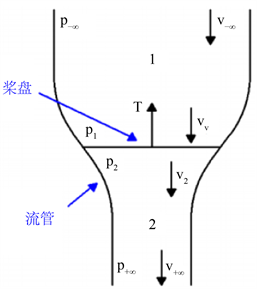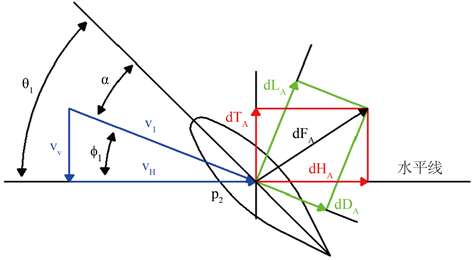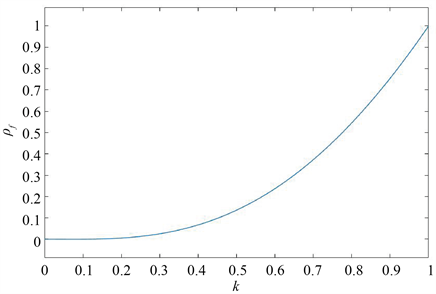#### 期刊菜单

DOI: 10.12677/JAST.2022.102002, PDF, HTML, XML, 下载: 59  浏览: 388  科研立项经费支持

Abstract: It is easy for the blade tip of quadrotor to have defect or fracture in real application. This would directly affect the generated thrust of quadrotor such that the flight quality or performance of quadrotor may be degraded. Therefore, the effect of blade tip defect, which is actually one kind of actuator faults, is analyzed from mechanism in this paper, and then the real generated thrust is calculated accordingly. Finally, the model of blade tip defect fault of quadrotor is obtained, which provides a foundation for designing the flight control scheme for quadrotor.

1. 引言

2. 基本知识

2.1. 桨叶动量定理

1) 穿过圆盘的空气流管与外部气流不存在相互作用；

2) 螺旋桨包含了无限多个桨叶元素；

3) 圆盘厚度无限薄；

4) 通过圆盘的气流的垂直速度是连续的；

5) 空气不可压缩。

${T}_{MT}=A\left({p}_{1}-{p}_{2}\right)={\stackrel{˙}{m}}_{A}\left({v}_{-\infty }-{v}_{+\infty }\right)={\rho }_{A}A{v}_{1}\left({v}_{-\infty }-{v}_{+\infty }\right)$ (1)Figure 1. The diagram of blade model in momentum theory

2.2. 叶素理论

$\begin{array}{l}\text{d}{L}_{BET}=\frac{1}{2}{\rho }_{A}{v}_{H}^{2}{C}_{L}c\text{d}r\\ \text{d}{D}_{BET}=\frac{1}{2}{\rho }_{A}{v}_{H}^{2}{C}_{D}c\text{d}r\end{array}$ (2)

${C}_{L}=a\alpha =a\left({\theta }_{I}-{\varphi }_{I}\right)$

${\theta }_{I}={\theta }_{0}-{\theta }_{tw}\frac{r}{R}$Figure 2. The diagram of force analysis on the blade section

${\varphi }_{I}=\frac{{v}_{V}}{{v}_{H}}$

$\text{d}{L}_{BET}=\frac{1}{2}{\rho }_{A}{v}_{H}^{2}a\left({\theta }_{0}-{\theta }_{tw}\frac{r}{R}-\frac{{v}_{V}}{{v}_{H}}\right)c\text{d}r$ (3)

$\text{d}{T}_{BET}=\text{d}{L}_{BET}\mathrm{cos}{\varphi }_{I}-\text{d}{D}_{BET}\mathrm{sin}{\varphi }_{I}\approx \text{d}{L}_{BET}$ (4)

$\text{d}{T}_{BET}$$\left[0,R\right]$ 区间求积分即可得到单片桨叶所能产生的推力 ${T}_{BET}$，具体为：

${T}_{BET}={\int }_{0}^{R}\frac{\text{d}{T}_{BET}}{\text{d}r}\text{d}r={\int }_{0}^{R}\frac{1}{2}{\rho }_{A}{v}_{H}^{2}a\left({\theta }_{0}-{\theta }_{tw}\frac{r}{R}-\frac{{v}_{V}}{{v}_{H}}\right)c\text{d}r$ (5)

3. 桨尖缺损故障建模

${v}_{H}=\omega r$

$\begin{array}{l}{p}_{-\infty }+\frac{1}{2}{\rho }_{A}{v}_{-\infty }^{2}={p}_{1}+\frac{1}{2}{\rho }_{A}{v}_{V}^{2}\\ {p}_{2}+\frac{1}{2}{\rho }_{A}{v}_{2}^{2}={p}_{+\infty }+\frac{1}{2}{\rho }_{A}{v}_{+\infty }^{2}\end{array}$

${v}_{1}=\frac{{v}_{+\infty }+{v}_{-\infty }}{2}$

${v}_{I}={v}_{1}-{v}_{-\infty }=\frac{{v}_{+\infty }-{v}_{-\infty }}{2}$

$T=2{\rho }_{A}A{v}_{1}{v}_{I}=2{\rho }_{A}A{v}_{V}^{2}$

$T=\frac{mg}{4}$

${v}_{I}={v}_{V}=\frac{1}{2}\sqrt{\frac{mg}{2{\rho }_{A}A}}$

$\lambda =\frac{{v}_{I}}{\omega R}$

${v}_{V}=\lambda \omega R$ (6)

$\begin{array}{c}{T}_{BET}\approx \frac{{\rho }_{A}ac}{2}{\int }_{0}^{kR}\left({\theta }_{0}{v}_{H}^{2}-{\theta }_{tw}\frac{r}{R}{v}_{H}^{2}-{v}_{V}{v}_{H}\right)\text{d}r\\ =\frac{{\rho }_{A}ac{\omega }^{2}{R}^{3}}{2}\left(\frac{{\theta }_{0}{k}^{3}}{3}-\frac{{\theta }_{tw}{k}^{4}}{4}-\frac{\lambda {k}^{2}}{2}\right)\end{array}$

$\begin{array}{c}{T}_{f}=2{T}_{BET}\approx {\rho }_{A}ac{\omega }^{2}{R}^{3}\left(\frac{{\theta }_{0}{k}^{3}}{3}-\frac{{\theta }_{tw}{k}^{4}}{4}-\frac{\lambda {k}^{2}}{2}\right)\\ ={\rho }_{A}ac{\omega }^{2}{R}^{3}{\rho }_{f}\left(\frac{{\theta }_{0}}{3}-\frac{{\theta }_{tw}}{4}-\frac{\lambda }{2}\right)\end{array}$

${\rho }_{f}=\frac{\left(\frac{{\theta }_{0}{k}^{3}}{3}-\frac{{\theta }_{tw}{k}^{4}}{4}-\frac{\lambda {k}^{2}}{2}\right)}{\left(\frac{{\theta }_{0}}{3}-\frac{{\theta }_{tw}}{4}-\frac{\lambda }{2}\right)}$

${T}_{f}={\rho }_{f}{T}_{n}$

4. 算例

${\theta }_{0}=0.67\text{\hspace{0.17em}}\text{rad},\text{\hspace{0.17em}}{\theta }_{tw}=0.29\text{\hspace{0.17em}}\text{rad},\text{\hspace{0.17em}}\lambda =0.05$Figure 3. The curve of ${\rho }_{f}$ with respect to k

5. 结论

NOTES

*通讯作者。

  刘小雄, 章卫国, 李广文, 等. 无人机自主编队飞行控制的技术问题[J]. 电光与控制, 2007, 13(6): 28-31.  姜斌, 冒泽慧, 杨浩, 张友民. 控制系统的故障诊断与故障调节[M]. 北京: 国防工业出版社, 2009.  周东华, 孙优贤. 控制系统的故障检测与诊断技术[M]. 北京: 清华大学出版社, 1994.  邹泉. 直升机旋翼桨叶系统动力学分析[D]: [硕士学位论文]. 西安: 西安电子科技大学, 2010.  Naidoo, Y., Stopforth, R. and Bright, G. (2011) Quad-Rotor Unmanned Aerial Vehicle Helicopter Modelling & Control. International Journal of Advanced Robotic Systems, 8, 45-55. https://doi.org/10.5772/45710  Bresciani, T. (2008) Modelling, Identification and Control of a Quadrotor Helicopter. MSc Thesis, Lund University.  Johnson, W. (1980) Helicopter Theory. Princeton University Press, New Jersey.  曹义华. 直升机机动飞行旋翼的气动力模拟[J]. 航空学报, 1999, 20(1): 39-42.  王适存, 主编. 直升机空气动力学[M]. 北京: 航空专业教材编审组, 1985.  徐国华, 招启军. 直升机旋翼计算流体力学的研究进展[J]. 南京航空航天大学学报, 2003, 53(3): 338-344.